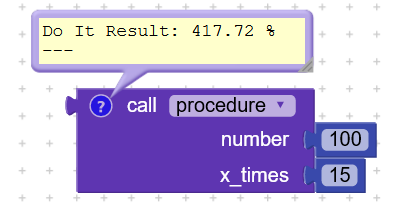Finding the result by adding on each time (%)

I am giving an example, in the form of 15 repetitions, the number of 100 is increasing by 10%.

1. =110
2. = 121
3. = 124.1

and finally 15. = ?

I’m a little confused how we can do this.

sample
100+10=110
110+11=121
121+12.1=133.1
133+…